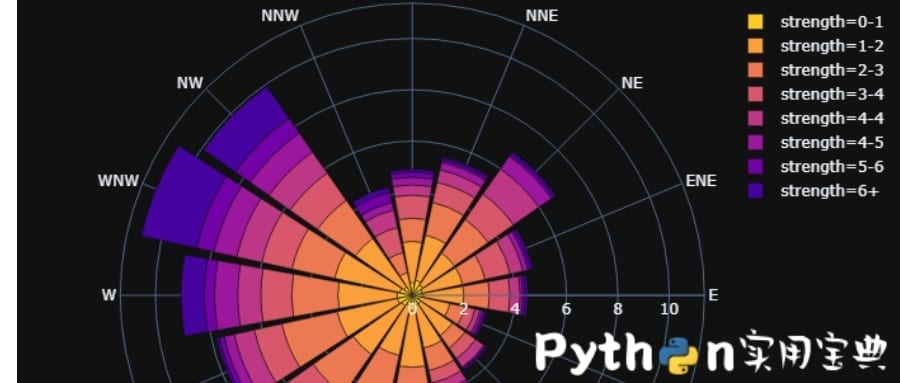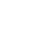# 精美的数据分析图!教你使用Python的Plotly库

plotly 是目前已知的Python最强绘图，它比上次我们讲的echarts还强大许多许多，它的绘制通过生成一个web页面得到，而且支持调整图像大小后保存，而且还能支持可动态调节的页面，方便python web端的开发。

### 一、安装

Windows环境下打开Cmd(开始—运行—CMD)，苹果系统环境下请打开Terminal(command+空格输入Terminal)，准备开始输入命令安装依赖。

`pip install plotly`

### 二、使用例子

data_frame：数据，需要以DataFrame格式。

color: 可指定每一列的特定颜色。

color_continuous_scale: 构建连续的颜色

https://plotly.github.io/plotly.py-docs/generated/plotly.express.parallel_categories.html

```# 文件命名为：test.py
import plotly.express as px
tips = px.data.tips()
fig = px.parallel_categories(tips, color="size", color_continuous_scale=px.colors.sequential.Inferno)
fig.show()```

```import plotly.express as px
gapminder = px.data.gapminder()
fig = px.scatter(gapminder.query("year==2007"), x="gdpPercap", y="lifeExp", size="pop", color="continent",
hover_name="country", log_x=True, size_max=60)
fig.show()```

### 三、保存

```import plotly.offline as offline
offline.plot(figure_or_data = fig, image = 'png', image_filename='plot_image', output_type='file', image_width=800, image_height=600, validate=False) ```

```# 文件命名为：test.py
import plotly.express as px
import plotly.offline as offline
tips = px.data.tips()
fig = px.parallel_categories(tips, color="size", color_continuous_scale=px.colors.sequential.Inferno)
fig.show()

offline.plot(figure_or_data = fig, image = 'png', image_filename='plot_image',
output_type='file', image_width=800, image_height=600, validate=False)```

### 四、其他功能

1. 共享：如果你希望能和其他人共享图片，而自己又不会做web开发，没关系，plotly也提供了解决方案，这个时候我们需要安装一个新的包叫chart_studio，然后使用官方给定的API进行在线绘制，就能保存到你的plotly个人网页中了！ 详细可见官方教程
2. 添加背景图：你还可以给你的图片添加背景图！而且操作非常简单。
3. 添加你的LOGO：添加你个人独特的LOGO也是支持的！

https://plot.ly/python/images/ 你可以找到这2和3的相应教程！

​Python实用宝典 (pythondict.com)